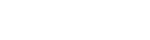# Interquartile Distance

Upvote
2
Downvote
Save
Mark Completed
Have you seen this question before?

Given an array of unsorted random numbers denoted `nums` write a function `find_iqr` to find the interquartile distance.

The interquartile distance is defined by subtracting the first quartile
from the third quartile.

Example:

Input:

``````nums = [3,4,9,12,14,239]
``````

Output:

``````def find_iqr(nums) -> 10
``````
Next question: Marked Lists
.....